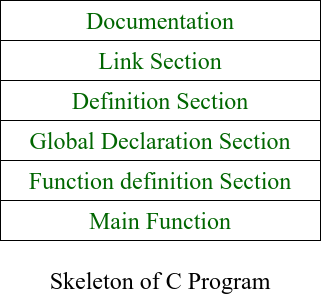Related Articles
Structure of C++ Program
• Difficulty Level : Basic
• Last Updated : 15 Dec, 2020

The C++ program is written using a specific template structure. The structure of the program written in C++ language is as follows:### Documentation Section:

• This section comes first and is used to document the logic of the program that the programmer going to code.
• It can be also used to write for purpose of the program.
• Whatever written in the documentation section is the comment and is not compiled by the compiler.
• Documentation Section is optional since the program can execute without them. Below is the snippet of the same:

## C++

 `/*     This is a C++ program to find the``        ``factorial of a number`` ` `    ``The basic requirement for writing this``    ``program is to have knowledge of loops`` ` `    ``To find the factorial of number``    ``iterate over range from number to one``*/`

The linking section contains two parts:

• Generally, a program includes various programming elements like built-in functions, classes, keywords, constants, operators, etc. that are already defined in the standard C++ library.
• In order to use such pre-defined elements in a program, an appropriate header must be included in the program.
• Standard headers are specified in a program through the preprocessor directive #include. In Figure, the iostream header is used. When the compiler processes the instruction #include<iostream>, it includes the contents of the stream in the program. This enables the programmer to use standard input, output, and error facilities that are provided only through the standard streams defined in <iostream>. These standard streams process data as a stream of characters, that is, data is read and displayed in a continuous flow. The standard streams defined in <iostream> are listed here.

#include<iostream>

Namespaces:

• A namespace permits grouping of various entities like classes, objects, functions, and various C++ tokens, etc. under a single name.
• Any user can create separate namespaces of its own and can use them in any other program.
• In the below snippets, namespace std contains declarations for cout, cin, endl, etc. statements.
`using namespace std;`
• Namespaces can be accessed in multiple ways:
• using namespace std;
• using std :: cout;

### Definition Section:

• It is used to declare some constants and assign them some value.
• In this section, anyone can define your own datatype using primitive data types.
• In  #define is a compiler directive which tells the compiler whenever the message is found replace it with “Factorial\n” .
• typedef int K; this statement telling the compiler that whenever you will encounter K replace it by int and as you have declared k as datatype you cannot use it as an identifier.

### Global Declaration Section:

• Here the variables and the class definitions which are going to be used in the program are declared to make them global.
• The scope of the variable declared in this section lasts until the entire program terminates.
• These variables are accessible within the user-defined functions also.

### Function Declaration Section:

• It contains all the functions which our main functions need.
• Usually, this section contains the User-defined functions.
• This part of the program can be written after the main function but for this, write the function prototype in this section for the function which for you are going to write code after the main function.

## C++

 `// Function to implement the``// factorial of number num``int` `factorial(k& num)``{``    ``// Iterate over the loop from``    ``// num to one``    ``for` `(k i = 1; i <= num; i++) {``        ``fact *= i;``    ``}`` ` `    ``// Return the factorial calculated``    ``return` `fact;``}`

### Main Function:

• The main function tells the compiler where to start the execution of the program. The execution of the program starts with the main function.
• All the statements that are to be executed are written in the main function.
• The compiler executes all the instructions which are written in the curly braces {} which encloses the body of the main function.
• Once all instructions from the main function are executed control comes out of the main function and the program terminates and no further execution occur.

Below is the program to illustrate this:

## C++

 `// Documentation Section``/*  This is a C++ program to find the``    ``factorial of a number``    ``The basic requirement for writing this``    ``program is to have knowledge of loops``    ``To find the factorial of a number``    ``iterate over the range from number to 1``*/`` ` `// Linking Section``#include ``using` `namespace` `std;`` ` `// Defination Section``#define msg "FACTORIAL\n"``typedef` `int` `k;`` ` `// Global Declaration Section``k num = 0, fact = 1, storeFactorial = 0;`` ` `// Function Section``k factorial(k& num)``{``    ``// Iterate over the loop from``    ``// num to one``    ``for` `(k i = 1; i <= num; i++) {``        ``fact *= i;``    ``}`` ` `    ``// Return the factorial``    ``return` `fact;``}`` ` `// Main Function``int` `main()``{``    ``// Given number Num``    ``k Num = 5;`` ` `    ``// Function Call``    ``storeFactorial = factorial(Num);``    ``cout << msg;`` ` `    ``// Print the factorial``    ``cout << Num << ``"! = "``         ``<< storeFactorial << endl;`` ` `    ``return` `0;``}`
Output
```FACTORIAL
5! = 120
```
Want to learn from the best curated videos and practice problems, check out the C++ Foundation Course for Basic to Advanced C++ and C++ STL Course for the language and STL.  To complete your preparation from learning a language to DS Algo and many more,  please refer Complete Interview Preparation Course.
My Personal Notes arrow_drop_up